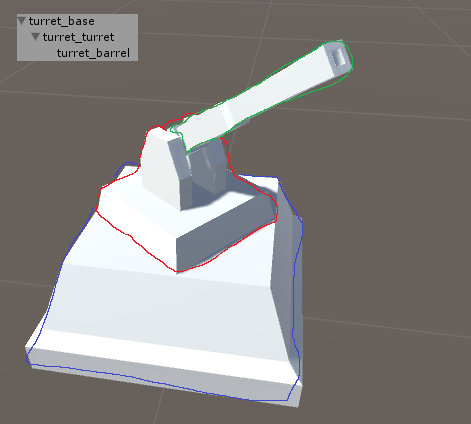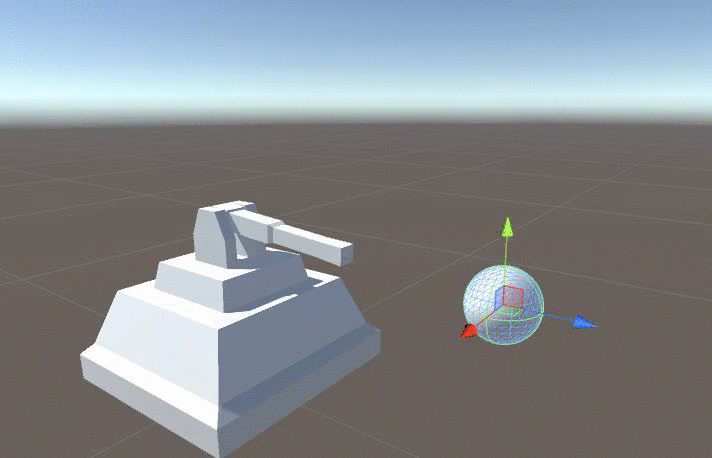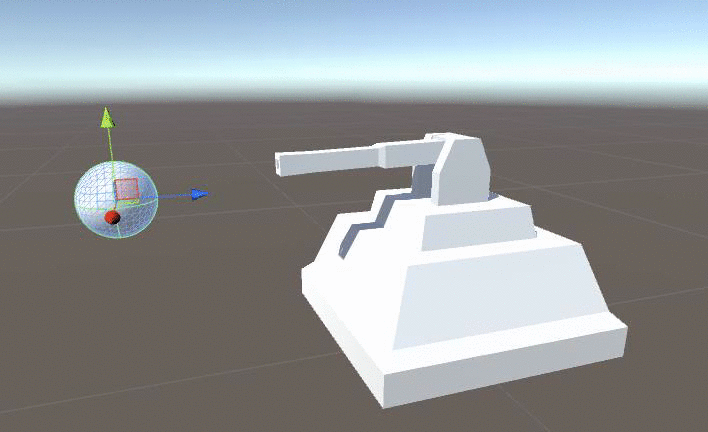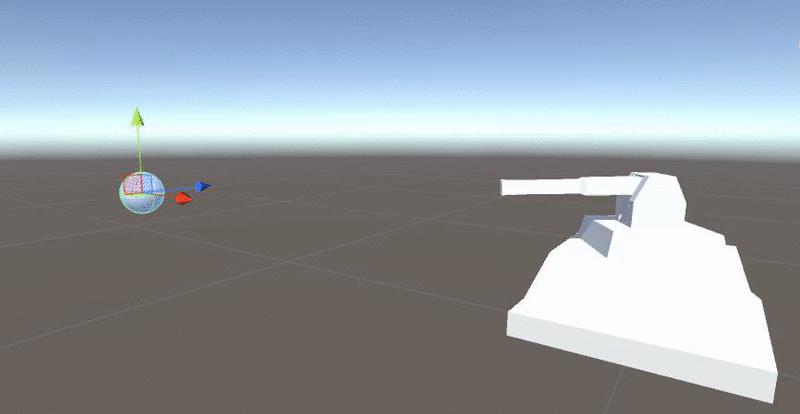# Unity's Quaternion.Lerp slows down when target is directly behind turret

Software: Unity v5.2.1f1 Personal

I am trying to achieve the effect of a turret in Unity. For this I have a turret consisting of 3 parts as well as a target. The problem is that whenever the target my turret is targeting has a z of < 0, the lerp speed is extremely slow. However, the further away the target is from x=0 (both positive and negative), the faster it will move. This effect only occurs when my target has a transform.position.z of < 0. When z > 0 the transform.position.x has no effect on the lerp speed. My setup is as follows:

The 3 turret parts are the base, turret and barrel.

The base is nothing more than a block for the turret to rest on. The turret is a block holding the barrel in place with base as his parent. The turret rotates around its y-axis to always face the target. The barrel has turret as his parent. The barrel rotates around its x-axis to always face the target. See the picture below for the end result.

Base: Blue; Turret: Red; Barrel: Green;The turret has the following code:

public class turretRotation : MonoBehaviour {

public Transform target;

void Update()
{
Vector3 relativePosition = target.position - transform.position;
relativePosition.y = 0;
Quaternion rotation = Quaternion.LookRotation(relativePosition, new Vector3(0, 1, 0));
transform.localRotation = Quaternion.Lerp(transform.localRotation, rotation, Time.deltaTime);
}
}


The barrel has the following code:

public class barrelRotation : MonoBehaviour {

public Transform target;
public float speed = 1;
Vector3 relPos;
Quaternion rot;

void Update () {
// Position of target relative to my position
relPos = (target.position - transform.position).normalized;

rot = Quaternion.LookRotation(relPos, new Vector3(0,1,0));

// Lerping localRotatiot > rot with speed: Time.deltaTime * speed (set to 1 by default)
transform.localRotation = Quaternion.Lerp(transform.localRotation, rot, Time.deltaTime *  speed);

// Resetting y- and z-EulerAngles to 0; This way only the x-EulerAngle will be affected.
// The y rotation is inherited from the parent (turret_turret)
transform.localEulerAngles = new Vector3(transform.localEulerAngles.x, 0, 0);
}
}


In this picture the Lerp is working as intended; Note target.transform.z > 0In this picture the target has a z = -5 and x = 0; pay particular attention to the Lerp speedIt eventually gets there, but it takes a very long time. For some reason, the further away the target is from x=0, the faster the Lerp speed. Note: Still slower than intended

In this picture the target has a z = -5 and x = -10So for some reason when target.transform.z has a negative value, the higher the distance from target.transform.x to x=0 the higher the Lerp speed.

I've done a fair bit of searching and came across a post on answers.unity.com describing a similair problem; Unfortunately, no reply. http://answers.unity3d.com/questions/1084067/lerp-problem-when-target-is-behind.html

So my question is: "Why is this happening and more importantly, how can I fix this?"

Any help would be much appreciated!

• Because it depends on distance, there might be something wrong with normalization - maybe re-phrasing the code(using temp variable, or Normalize() instead of .normalized) would help? – wondra Oct 20 '15 at 20:24
• Thank you for your reply, I've tried using Vector3.Normalize() instead of .normalized, but to no avail. I am not sure what you mean with using temp variables though. Could you further clarify? Again thanks for the assistance. – Stijn van der Pol Oct 20 '15 at 20:43
• Assigning the result (loc-target) to local variable, normalizing it on next line, just to be super-safe the issue is not normalization. – wondra Oct 20 '15 at 21:05
• Ah, I've tried it, but unfortunately the problem still persists. – Stijn van der Pol Oct 20 '15 at 21:17

## 1 Answer

Taking a look at your barrel rotation function...

relPos = (target.position - transform.position).normalized;
rot = Quaternion.LookRotation(relPos, new Vector3(0,1,0));


relPos is in world space, so rot is an absolute rotation (ie. relative to the world coordinate frame)

transform.localRotation = Quaternion.Lerp(
transform.localRotation,
rot,
Time.deltaTime *  speed);


transform.localRotation is relative to the parent's coordinate space.

So this Lerp is blending between a relative orientation and an absolute one.

When you use Lerp this way, the speed or increment each frame is proportional to the difference between the start & end values. (This adds a nice bit of slowing as the rotation approaches the target compared to Quaternion.RotateTowards() which enforces a particular speed)

Assuming your barrel is already pointing somewhat near your target...

• When the parent's rotation is very similar to the world coordinate frame, the difference between transform.localRotation and rot will tend to be small (slow tracking).

• When the parent's is rotated a lot relative to the world coordinate frame, the difference between transform.localRotation and rot will tend to be very large (fast tracking).

So you'll want to make sure you're using apples-to-apples comparisons when Lerping - either work entirely in local space (eg. with transform.parent.InverseTransformDirection(relPos) or Quaternion.Inverse(transform.parent.rotation) ) or entirely in world space.

• Thank you for your reply and thorough explanation. I understand my mistake and I added the line relPos = transform.parent.InverseTransformDirection(relPos);. It works perfectly now. Again, thank you very much. – Stijn van der Pol Oct 21 '15 at 12:51